# Total Excel Converter X

Convert XLS, XLSX, ODS, XML spreadsheets in batch through web-servers.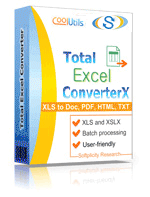Windows
2000/2003/Vista/7/8/10

The Total Excel Converter X builds on the award-winning functionality of the Total Excel Converter by adding support for web-based, server-oriented activities. It easily and accurately converts Excel to Word (both Doc and DocX), PDF, HTML, Access, TXT, ODT, ODS, XML, SQL, CSV, Lotus, DBF, TEX, DIFF, SYLK, and LaTeX files . The Total Excel Converter X also includes support for ActiveX and command line access.

Due to its advanced, web-based design the Total Excel ConverterX has no GUI or interrupting messages. It can convert your XLS files quietly and automatically. Convert single Excel sheets, or work with large batches of files; the choice is yours. Either way, get ready to save lots of time!

• Able to work with XLS, XLSX and OpenOffice formats (.odt, .ods), .xml, .sql, wk2, wks, .dbf, .tex, .dif as source files.
• Includes support for ActiveX, allowing for integration into web interfaces; make the converter a part of any existing web application!
• Adding Total Folder Monitor lets you track activity in any folder and auto-run Total Excel Converter X functions when new XLS files arrive. Learn more here.

The Total Excel Converter X is a powerful console tool without a cumbersome GUI. Designed to help you perform back-end conversions automatically, this powerful utility is backed by a decade's worth of testing and user feedback. Try it today by downloading our free trial version.

(includes 30 day FREE trial)

(only \$550.00)

## Examples of Total Excel ConverterX

### Convert excel files With TotalExcelConverterX and .NET

```string src="C:\\test\\Source.xlsx";
string dest="C:\\test\\Dest.PDF";

ExcelConverterX Cnv = new ExcelConverterX();
Cnv.Convert(src, dest, "-c PDF -log c:\\test\\Excel.log");

MessageBox.Show("Convert complete!");
```

### Convert Excel Files On Web Servers With Total Excel ConverterX

```dim C
Set C=CreateObject("ExcelConverter.ExcelConverterX")
C.Convert "c:\test\source.xlsx", "c:\test\dest.pdf", "-cPDF -log c:\test\Excel.log"
Response.Write C.ErrorMessage
set C = nothing
```
Example2 ASP: directly stream the resulting PDF
```dim C
Set C=CreateObject("ExcelConverter.ExcelConverterX")
Response.Clear
Response.BinaryWrite c.ConvertToStream("C:\www\ASP\Source.xlsx", "C:\www\ASP", "-cpdf  -log c:\html.log")
set C = nothing
```

### Convert Excel Spreadsheets by PHP With Total Excel ConverterX

```\$src="C:\\test\\test.XLS";
\$dest="C:\\test\\test.CSV";
\$c= new COM("ExcelConverter.ExcelConverterX");
\$c->convert(\$src,\$dest, "-c csv  -log c:\\test\\xls.log");
if (file_exists(\$dest)) echo "OK"; else echo "fail:".\$c->ErrorMessage;
```

### Convert Excel Spreadsheets By Ruby With Total Excel ConverterX

```require 'win32ole'
c = WIN32OLE.new('ExcelConverter.ExcelConverterX')

src="C:\\test\\test.xlsx";
dest="C:\\test\\test.pdf";

c.convert(src,dest, "-c PDF -log c:\\test\\Excel.log");

if not File.exist?(dest)
puts c.ErrorMessage
end

```

### Convert Excel Spreadsheets by Python With Total Excel ConverterX

```import win32com.client
import os.path

c = win32com.client.Dispatch("ExcelConverter.ExcelConverterX")

src="C:\\test\\test.xlsx";
dest="C:\\test\\test.pdf";

c.convert(src, dest, "-c PDF -log c:\\test\\Excel.log");

if not os.path.exists(file_path):
print(c.ErrorMessage)
```

### Convert Excel files With Pascal and Total Excel ConverterX

```uses Dialogs, Vcl.OleAuto;

var
c: OleVariant;
begin
c:=CreateOleObject('ExcelConverter.ExcelConverterX');
C.Convert('c:\test\source.xlsx', 'c:\test\dest.pdf', '-cPDF -log c:\test\Excel.log');
IF c.ErrorMessage<> Then
ShowMessage(c.ErrorMessage);
end;
```

### Convert Excel and ODS Files On Web Servers With Total Excel ConverterX

```var c = new ActiveXObject("ExcelConverter.ExcelConverterX");
c.Convert("C:\\test\\source.xlsx", "C:\\test\\dest.pdf", "-c PDF");
if (c.ErrorMessage!="")
```

### Convert Excel Spreadsheets by Perl With Total Excel ConverterX

```use Win32::OLE;

my \$src="C:\\test\\test.XLS";
my \$dest="C:\\test\\test.CSV";

my \$c = CreateObject Win32::OLE 'ExcelConverter.ExcelConverterX';
\$c->convert(\$src,\$dest, "-c csv  -log c:\\test\\xls.log");
print \$c->ErrorMessage if -e \$dest;
```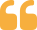### Total Excel Converter X Customer Reviews 2020

Rated 4.7/5 based on 20 customer reviews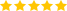I have tried many Excel to CSV converters, even all of free online services, but, they did not correctly converted my files. It is because, my Excel files contained Russian (Cyrillic alphabet) characters, and all other converters failed to represent Cyrillic characters correctly. Only Total Excel Converter did the job correctly, also Total Excel Converter has very simple easily understandable menu, it is very easy to navigate through menu."Zhandos Alpyssov www.ceravit.com

"It is very promising and good product. We have tested Excel to text and xls to doc conversions so far, it worked all right. Thank you for the support."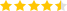Marcus Todsen

Updated Mon, 21 Oct 2019

(only \$550.00)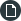Help (PDF)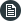Licenses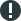Upgrades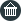Resellers